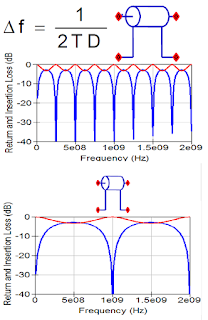## You need to test, we're here to help.

You need to test, we're here to help.

## 04 May 2020Figure 1. Ripple patterns commonly seen in S11 and S21.
When we look at plots of S-parameters, we can observe four classic patterns that affect the S11 reflection coefficient (aka. return loss) and the S21 transmission coefficient (aka. insertion loss): ripples, monotonic drop offs, broad dips and sharp dips. In this post, we’ll look into how the characteristics of the interconnect affect the ripple pattern, which will help you better understand your own measurements.

The ripples in S11 and sometimes in S21 in Figure 1 are due to reflections in the interconnect caused by impedance discontinuities. This phenomenon has been investigated in an earlier post, What S-parameters Reveal About Interconnects (Part III)Figure 2. Frequency interval of ripple in S11 (blue) is related to round-trip delay due to length of the interconnect.
Physically, the longer the interconnect, and hence the greater the round trip delay time (2 x TD) of the interconnect, the shorter the frequency interval (Df) between dips. This effect repeats at frequencies where the round-trip phase shift is a multiple of 1 cycle. For the interconnect used in the lower plot in Figure 2, a length of three inches results in a 1 ns round trip time delay and a 1 GHz frequency interval between dips.

Now that we have seen that the frequency interval of the ripple depends on the length of the interconnect, you might then ask: “what affects the amplitude of the S11 ripple?” It turns out that the magnitude of the impedance discontinuities causing the reflection determines the amplitude of the ripple in the reflection coefficient S11, as shown in Figure 3.

The amplitude of the ripple in S11 depends on the difference from the port impedance of the impedance of the interconnect. Observe that the greater the difference from the port and interconnect impedances , the greater (less negative in dB) the magnitude of the ripple. An interconnect impedance of 40 ohms, a 10 ohm difference, produces -14 dB of ripple in the top graph of Figure 3, while a 30 ohm difference increases the ripple magnitude to-4 dB in the lower graph.Figure 3. The magnitude of the S11 ripple is related to the impedance difference across the interconnect.
The ripple in S21 (the red traces in Figure 3) is synchronous with that in S11 (the blue traces), and that is because they are related: ripple in S11 is visible in S21 when S11 is greater than -13 dB, as is the case in the bottom graph in Figure 3. This is explained in What S-parameters Reveal About Interconnects (Part II).

In the next post in this series, we'll discuss the monotonic drop offs seen in S-parameters. In the meantime, watch the on-demand webinar Reading S-Parameters Like a Book by Dr. Eric Bogatin, Signal Integrity Evangelist, for a more in-depth treatment of this topic.